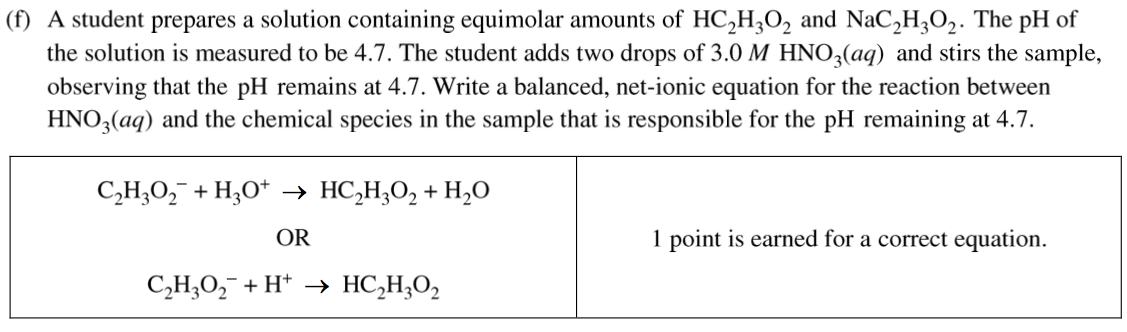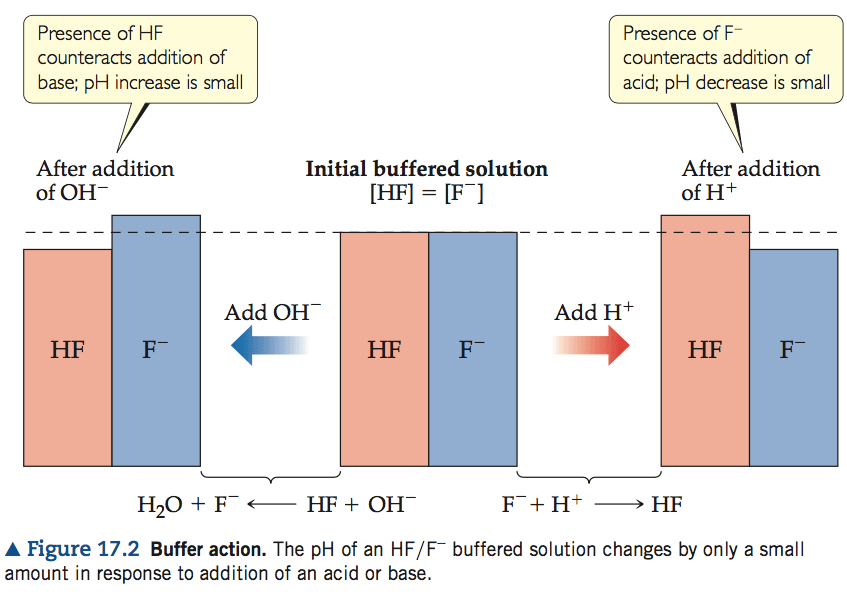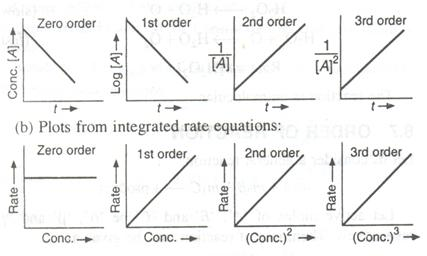# Question 1 (a)# Question 1 (c)# Question 1 (d)# Question 1 (f)# Question 2 (e)# Question 2 (f)# Question 3 (d)# Question 5 (b)

![Zeroth Order First Order Second Order Differential rate law Concentration vs. time Integrated rate law Straight-line plot to determine rate constant Relative rate vs. concentration Half-life Units of k, rate constant Rate = o o o o ALA] At Time Slope = —k Time Rate — o o ALA] = MA] At Time o o —kt or Slope = —k Time [A], M Rate, M/S o o o o 2 3 M Rate, M/s 2k 2 3 Rate = 2 3 A[A] = At Time Slope = k Time Rate, M/S 4 9 2 3 0.693 l/s ](./media/image22.png)# Question 6 (a)# Question 7 (a)

• Since your buret is graduated to 0.1 mL, you will read your buret to 0.01 ml. The second decimal place is an estimate, but should be recorded.